Download Presentation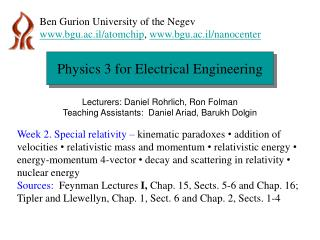Physics 3 for Electrical Engineering

# Physics 3 for Electrical Engineering - PowerPoint PPT Presentation

Ben Gurion University of the Negev. www.bgu.ac.il/atomchip , www.bgu.ac.il/nanocenter. Physics 3 for Electrical Engineering. Lecturers: Daniel Rohrlich, Ron Folman Teaching Assistants: Daniel Ariad, Barukh Dolgin.I am the owner, or an agent authorized to act on behalf of the owner, of the copyrighted work described.
Download Presentation## Physics 3 for Electrical Engineering

An Image/Link below is provided (as is) to download presentation

Download Policy: Content on the Website is provided to you AS IS for your information and personal use and may not be sold / licensed / shared on other websites without getting consent from its author.While downloading, if for some reason you are not able to download a presentation, the publisher may have deleted the file from their server.

- - - - - - - - - - - - - - - - - - - - - - - - - - E N D - - - - - - - - - - - - - - - - - - - - - - - - - -
Presentation Transcript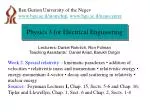Ben Gurion University of the Negev

www.bgu.ac.il/atomchip,www.bgu.ac.il/nanocenter

Physics 3 for Electrical Engineering

Lecturers: Daniel Rohrlich, Ron Folman

Teaching Assistants: Daniel Ariad, Barukh Dolgin

Week 2. Special relativity – kinematic paradoxes • addition of velocities • relativistic mass and momentum • relativistic energy • energy-momentum 4-vector • decay and scattering in relativity • nuclear energy

Sources: Feynman Lectures I, Chap. 15, Sects. 5-6 and Chap. 16;

Tipler and Llewellyn, Chap. 1, Sect. 6 and Chap. 2, Sects. 1-4You go out into space for one year at 0.9 of the speed of light. When you come back, how old will your twin brother be? Is there a paradox here? How would you solve it?Grumpy lives in Haifa; Dumpy and Jump live in Tel Aviv. They are identical triplets with synchronized watches. One day, Jump takes a high-speed (v = c/2) train to visit Grumpy.

Grumpy and Dumpy expect Jump’s watch to run slow during the train ride. But Jump expects their watches to run slow! He reasons, “When the train left the station, I saw that my watch was still synchronized with Dumpy’s. So it was still synchronized with Grumpy’s watch. Now I am sitting and they are moving fast relative to me! So their watches must run slower than mine!”

Who is right???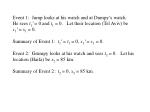Event 1: Jump looks at his watch and at Dumpy’s watch.

He sees t1′ = 0 and t1 = 0. Let their location (Tel Aviv) be

x1′ = x1 = 0.

Summary of Event 1: t1′ = t1 = 0, x1′ = x1 = 0.

Event 2: Grumpy looks at his watch and sees t2 = 0. Let his location (Haifa) be x2 = 85 km.

Summary of Event 2 : t2 = 0, x2 = 85 km.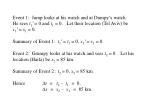Event 1: Jump looks at his watch and at Dumpy’s watch.

He sees t1′ = 0 and t1 = 0. Let their location (Tel Aviv) be

x1′ = x1 = 0.

Summary of Event 1: t1′ = t1 = 0, x1′ = x1 = 0.

Event 2: Grumpy looks at his watch and sees t2 = 0. Let his location (Haifa) be x2 = 85 km.

Summary of Event 2 : t2 = 0, x2 = 85 km.

Hence ∆t = t2 – t1 = 0,

∆x = x2 – x1 = 85 km.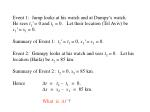Event 1: Jump looks at his watch and at Dumpy’s watch.

He sees t1′ = 0 and t1 = 0. Let their location (Tel Aviv) be

x1′ = x1 = 0.

Summary of Event 1: t1′ = t1 = 0, x1′ = x1 = 0.

Event 2: Grumpy looks at his watch and sees t2 = 0. Let his location (Haifa) be x2 = 85 km.

Summary of Event 2 : t2 = 0, x2 = 85 km.

Hence ∆t = t2 – t1 = 0,

∆x = x2 – x1 = 85 km.

What is ∆t′ ?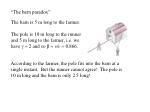The barn is 5 m long to the farmer.

The pole is 10 m long to the runner and 5 m long to the farmer, i.e. we have g = 2 and so b = v/c = 0.866.

According to the farmer, the pole fits into the barn at a single instant. But the runner cannot agree! The pole is 10 m long and the barn is only 2.5 long!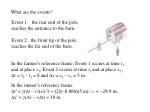What are the events?

Event 1: the rear end of the pole reaches the entrance to the barn.

Event 2: the front tip of the pole reaches the far end of the barn.

In the farmer’s reference frame, Event 1 occurs at time t1 and at place x1; Event 2 occurs at time t2 and at place x2 ;

Dt = t2 – t1 = 0 and Dx = x2 – x1= 5 m.

In the runner’s reference frame:

Dt′ = g(Dt – vDx/c2) = (2)(-0.866)(5 m) / c = -28.9 ns,

Dx′ = g(Dx – vDt) = 10 m.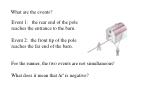What are the events?

Event 1: the rear end of the pole reaches the entrance to the barn.

Event 2: the front tip of the pole reaches the far end of the barn.

For the runner, the two events are not simultaneous!

What does it mean that Dt′ is negative?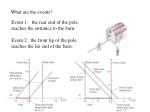What are the events?

Event 1: the rear end of the pole reaches the entrance to the barn.

Event 2: the front tip of the pole reaches the far end of the barn.Further applications of Lorentz transformations

• Dx′ = g(Dx – vDt)
• Dy′ = Dy
• Dz′ = Dz
• Dt′ = g(Dt – vDx/c2)

How do we derive

time dilation from the Lorentz transformations?cDt/2

L

cDt/2

vDt

If Alice (primed system) moves along the x-axis with velocity v relative to Bob (unprimed system), then

• Dx′ = g(Dx – vDt)
• Dy′ = Dy
• Dz′ = Dz
• Dt′ = g(Dt – vDx/c2)

How do we derive

time dilation from the Lorentz transformations?cDt/2

L

cDt/2

vDt

If Alice (primed system) moves along the x-axis with velocity v relative to Bob (unprimed system), then

• Dx′ = g(Dx – vDt)
• Dy′ = Dy
• Dz′ = Dz
• Dt′ = g(Dt – vDx/c2)

For Alice, the clock stays in one place. Hence Dx′ = 0.cDt/2

L

cDt/2

vDt

If Alice (primed system) moves along the x-axis with velocity v relative to Bob (unprimed system), then

• Dx′ = g(Dx – vDt) Hence Dx = vDt
• Dt′ = g(Dt – vDx/c2)= g(Dt – v2Dt/c2) = g(Dt/g2 ) = Dt/g

0 =

For Alice, the clock stays in one place. Hence Dx′ = 0.Further applications of Lorentz transformations

• Dx′ = g(Dx – vDt)
• Dy′ = Dy
• Dz′ = Dz
• Dt′ = g(Dt – vDx/c2)

How do we derive

length contraction from the Lorentz transformations?If the runner (primed system) moves along the x-axis with velocity v relative to the farmer (unprimed system), then

• Dx′ = g(Dx – vDt)
• Dy′ = Dy
• Dz′ = Dz
• Dt′ = g(Dt – vDx/c2)

How do we derive

length contraction from the Lorentz transformations?If the runner (primed system) moves along the x-axis with velocity v relative to the farmer (unprimed system), then

• Dx′ = g(Dx – vDt)
• Dy′ = Dy
• Dz′ = Dz
• Dt′ = g(Dt – vDx/c2)

For the farmer, Dx = L and Dt = 0.

For the runner, Dx′ = L′.If the runner (primed system) moves along the x-axis with velocity v relative to the farmer (unprimed system), then

• Dx′ = g(Dx – vDt) = gL
• Dy′ = Dy
• Dz′ = Dz
• Dt′ = g(Dt – vDx/c2)

L′ =

For the farmer, Dx = L and Dt = 0.

For the runner, Dx′ = L′.ux= dx/dt ; uy= dy/dt ; uz= dz/dt

ux′= dx′/dt′ ; uy′= dy′/dt′ ; uz′= dz′/dt′

For inertial reference frames moving with relative velocity v along the x–axis,

ux′= (ux–v)/(1–vux/c2)

uy′= uy /g(1–vux/c2)

uz′= uz /g(1–vux/c2)

Thus you can never surpass the speed of light e.g. by shooting a 0.9c bullet from a 0.9c jet:

(0.9c+0.9c)/[1+(0.9c) · (0.9c)/c2] = (1.80/1.81)c = 0.9945cDerivation: apply the Lorentz transformations to the differentials,

and substitute them into ux′, uy′ and uz′ to obtainRelativistic mass and momentum

We now move from kinematics (pure description of motion) to dynamics (description of forces).

We will discover that if momentum (mv) is conserved in one inertial reference frame, it is not conserved in another!

But conservation of momentum is a law of physics. And Einstein postulated that the laws of physics are the same in all reference frames….y

uy

The blue particle has mass m and speed v and so does the red particle. In the center-of-mass frame, total momentum is 0 before and after the collision.

x

uy

uxy

uy

The blue particle has mass m and speed v and so does the red particle. In the center-of-mass frame, total momentum is 0 before and after the collision.

x

uy

ux

But now we go to a reference frame moving to the right with speed ux:y

uy

The blue particle has mass m and speed v and so does the red particle. In the center-of-mass frame, total momentum is 0 before and after the collision.

x

uy

y

ux

uy′

But now we go to a reference frame moving to the right with speed ux:

x

uy

uy′

uy′

uy

uy′

ux′ = 0y

uy′

x

uy

uy′

uy′

uy

uy′

ux′ = 0Since uy′ +uy′ ≠ 0, the y-component of momentum is not conserved…unless we redefine momentum!

y

uy′

x

uy

uy′

uy′

uy

uy′

ux′ = 0Since uy′ +uy′ ≠ 0, the y-component of momentum is not conserved…unless we redefine momentum!

Let’s try p = m f(v) v with f(0)=1.

y

uy′

x

uy

uy′

uy′

uy

uy′

ux′ = 0y

Boost left ux

Boost right ux

x

ux

y

uy"

uy′

y

x

ux"= 0

x

uy′

Boost right v = ux"

uy"

ux′ = 0uy"

uy"

uy"

uy′

vux"/ c2

y

uy" = – uy′

uy′

y

x

ux"= 0

x

uy′

Boost right v = ux"

uy"= – uy′

ux′ = 0So now if we assume p = m f(v) v, then

and in the limit uy"→ 0 we replace ux" with v to obtain

and therefore p = mγv .v/c .

gmv/ mv

v/cRelativistic energy

We accelerate a body using a force F = dp/dt :If we accelerate the body from rest to a final speed v, we find that the change in kinetic energy of the body is

In the limit c → ∞ the kinetic energy is mv2/2. But what

is the meaning of the huge “rest energy” mc2 ?Energy-momentum 4-vector

E = mγc2, so E and pc form a 4-vector u = (E, pc) and

The energy-momentum 4-vector transforms like any other 4-vectorunder Lorentz transformations.

Hence both the energy E and the momentum p are conserved in each inertial reference frame.Decay and scattering in relativityA fateful example

A heavy particle of mass M decays into two lighter particles of mass m. What is the velocity of each of the lighter particles? How much kinetic energy is released?

Let us treat this problem in the rest frame of the heavy particle.

Energy-momentum conservation:

(Mc2, 0) = (mγ1c2, mγ1v1c) + (mγ2c2, mγ2v2c) = (E1, p1c) + (E2, p2c)

Hence p1= – p2 and γ1= γ2 = γ from momentum conservation, and Mc2 = 2mγc2 from energy conservation. Therefore γ = M/2m and

since we get .So in the rest frame of the heavy particle, the lighter particles come out back to back with opposite velocities and equal speeds

There is no kinetic energy initially. The final kinetic energy is

More generally, if the heavy particle decays into several lighter particles of masses mi, the kinetic energy released isApplication: fission of U235 into Ba141 and Kr92 and also two neutrons yields how much kinetic energy?

mass (a.m.u.)

U235

Ba141

Kr92

n

Kinetic energy yield

= (235.043923 – 140.914411 – 91.926156 – 2 × 1.008665) a.m.u.

× (1.660539× 10–27 kg/a.m.u.) × (2.997925 × 108 m/s)2

= 2.77629 × 10–11 JOne gram of U235 contains 2.56 × 1021 atoms of U235, so fission of one gram of pure U235 yields (2.56 × 1021 ) × (2.78 × 10–11 J)

= 7.12 × 1010 J of kinetic energy.

By way of comparison, consider two cars, each with 1500 kg mass, at speed 90 km/hour = 25 m/s, in a head-on collision. The total kinetic energy lost in the collision is (3000 kg) × (25 m/s)2 = 1.875 × 106 J. So fission of one gram of U235 has the destructive power of about 40,000 such collisions.Relativistic scattering: another fateful example

Two deuterium (1H2) nuclei collide and produce a helium-3 (2He3) nucleus and a neutron. What are the velocities and energies of the helium nucleus and the neutron?The easiest way to solve this problem is to go to the “center-of-momentum” frame, i.e. the inertial frame in which the total momentum is zero:The easiest way to solve this problem is to go to the “center-of-momentum” frame, i.e. the inertial frame in which the total momentum is zero:In this case the problem reduces to the previous problem, with the initial mass M of the decaying particle replaced by the initial energy of the two deuterium nuclei divided by c2.What is the minimum amount of kinetic energy produced by this scattering process (in the center-of-momentum frame)?What is the minimum amount of kinetic energy produced by this scattering process (in the center-of-momentum frame)?

It is [2 × m(deuterium) – m(helium-3) – m(neutron)] × c2Note: In fission of uranium-235, the fraction of mass converted into energy is

In fusion of two deuterium nuclei to helium-4, it isNuclear energy: the curve of binding energy

Average binding energy ∆E/A vs. mass number ANuclear energy: the curve of average nucleon mass

m/A

Average mass of nucleon m/Avs. mass number A